# Thomson Scattering

The Thomson scattering describes photon scattering by free electrons in the classical electricity and magnetism. This is an approximation in the low energy limit where the quantum effect is no longer significant.

Let me first remind you of some fundamental equations of the classical electricity and magnetism involving the photon radiation. In the system with a charge density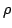and its current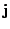(see the FIG. 1), the Maxwell equations give the electric scalar potential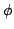and the vector potential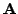as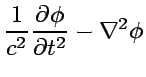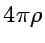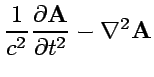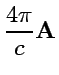(1)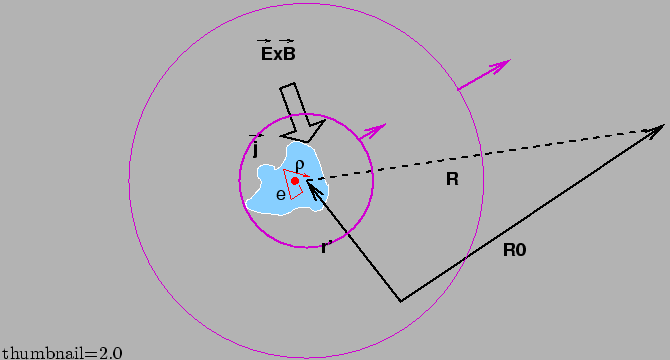The solutions of the equations above describe the famous delayed potential: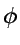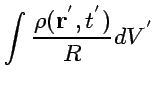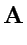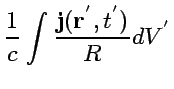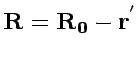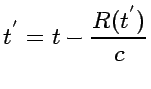(2)

When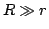, then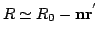, and the radiation fields are considered to be plane waves. Then only the vector potentialdescribes everything because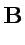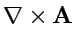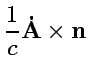(3)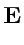(4)

where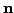is the unit vector parallel to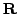. Consequently, the equations 4 reads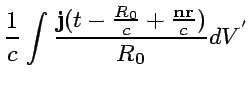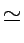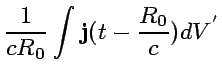(5)

The second equation above is valid when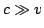. In this limit, the delayed time that determines the behavior ofis no longer related to the integration variables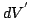. The integration in Eq. 5, therefore, can be made using the electric dipole expression,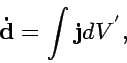(6)

and Eq. 4 gives the electric and magnetic fields radiated from the system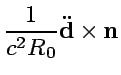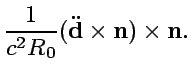(7)

Then the radiation intensity is obtained by the pointing vector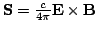as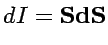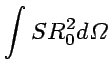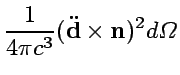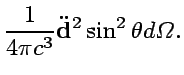(8)

Now let us consider the system that consist of an electron in the external field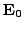and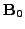. In the quantum picture, these fields correspond to a photon, which implies the interactions between a photon and an electron. When non-relativistic case,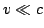, the electrical force is by far larger than the Lorentz force. Then the equation of motion of an electron gives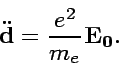(9)

Then the radiation intensity induced by the electron is obtained by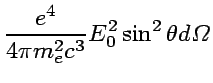(10)

The cross section, in this case, is defined by the energy flow described by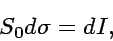(11)

where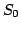is the strength of the external field pointing vector,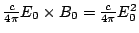. Then Eq. 10 gives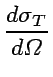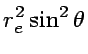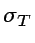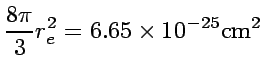(12)

where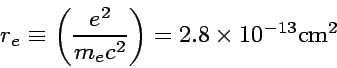(13)

is the classical electron radius. This type of scattering is called Thompson Scattering and the cross sections given by Eq.=12 gives the typical scale of the electro-magnetic interactions.

Subsections